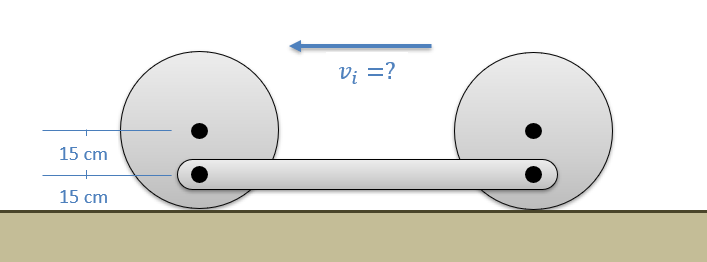﻿ Mechanics Map - Principle of Work and Energy for Rigid Bodies

# Principle of Work and Energy for Rigid Bodies

As stated in the previous section, the Principle of Work and Energy can be boiled down to the idea that the work done to a body will be equal to the change in energy of that body. Dividing energy into kinetic and potential energy pieces as we often do in dynamics problems, we arrive at the following base equation for the principle of work and energy.

 $W=\Delta KE + \Delta PE$

It is important to notice that unlike Newton's Second Law, the above equation is not a vector equation. It does not need to be broken down into components which can simplify the process, however, we only have a single equation and therefore can only solve for a single unknown which can limit the method.

Additionally, readers will see that these are the same base equations that were used for particles. The only difference with rigid bodies will be that there are additional types of work, kinetic energy, and potential energy that can be used in the overall equation.

## Solving Work and Energy Problems

To solve work and energy problems, we will need to first clearly define the initial state and the final state. Drawing a diagram that clearly shows each of these can be a useful first step.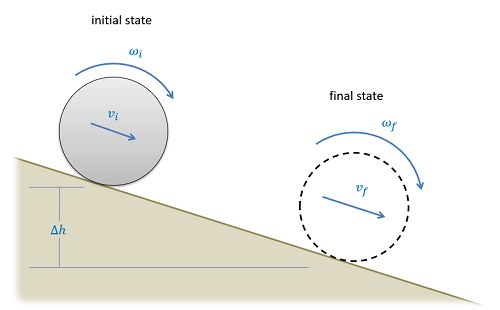A diagram showing the initial and final states in a work and energy problem can be a helpful first step in solving the problem.

After clearly defining the initial and final states, we will next need to start determining and plugging in the appropriate values to our work and energy equation. Add a work term for any external forces or moments acting on the system between the initial and final states (excluding gravity forces and spring forces). Determine the kinetic energy in the initial state and the final state and plug those into the equation, if there is a change in height plug in gravitational potential energy terms, and finally if there are any springs plug in any elastic potential energy terms.

After plugging in all the terms, you should hopefully have only a single unknown value that you can solve for. If you have more than one unknown, you may need to use kinematics or dependent motion analysis to relate the unknown velocities or displacements that you do have.

After putting all the terms into the work and energy equation and ensuring that there is only a single unknown, you will simply need to use algebra to solve for the unknown in the equation.

## Systems of Rigid Bodies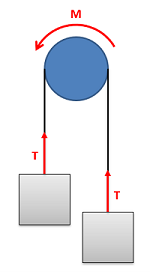When examining a system, only include the work done by external forces and moments. For example if the two boxes and the cable are our system, then the moment from the pulley is external, while the tension force in the cable is internal.

One of the ways to simplify work-energy problems involving multiple connected rigid bodies is to consider all the rigid bodies as one system. As discussed with particles internal forces are not counted in the work done to the system. If we include multiple rigid bodies in our system, then contact forces between the bodies as well as tension forces in cables that connect the bodies become internal forces, and we do not need to calculate the work done by them. The work in the equation will only need to account for external forces.

To use the work and energy principle with a system of particles, we would simply need to use the same equations as before, but ensure that the work is the work due to external forces, and ensure that we account for the kinetic and potential energies of all bodies included in our system.

## The Conservation of Energy

Just as with particles, if we have no external forces or moments acting on our system (ignoring gravity and spring forces) then we will have no work done to our system. If we eliminate the work term from the equations above, it will leave us with the following equations.

 $0=\Delta KE +\Delta PE$ $KE_i + PE_i =KE_f + PE_f$

Since the total energy does not change in these circumstances, these problems are known as conservation of energy problems. They are solved with much the same process as before. We will create a diagram of the initial and final state, determine energies in each of these states, and finally plug those values into our equation and solve for the unknown.

## Worked Problems:

### Question 1:

The turntable on a record player consists of a disk 12 inches in diameter with a weight of 5lbs. The motor accelerates the turntable from rest to it’s operating speed of 33.33 rpm in one rotation. What is the work done by the motor? What is the average torque the motor exerted?Image by Ron Clausen CC-BY-SA 4.0

### Question 2:

A 5 kg spherical ball with a radius of 5 cm is placed on a ramp as shown below. If the ball rolls without slipping, what is the expected velocity of the ball at the bottom of the ramp?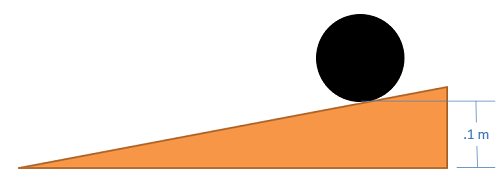### Question 3:

A system as shown below is used to passively slow the lowering of a gate. The gate can be approximated as a flat plate on its edge with a mass of 25 kg and a height of 2m. Assume the spring is unstretched as shown in the diagram.

• What would the angular velocity of the gate be without the spring?
• If we want to reduce the angular velocity at the bottom to 25% of its original value what should the spring constant be?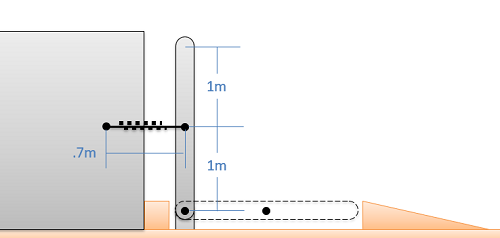### Question 4:

A lazy engineer is designing a robot to move things for him. He places a hub motor inside a 2.5 kg disk such that a couple moment of M=2.943 Nm is applied. If the attached package has a mass m=5 Kg and the coefficients of kinetic friction and static friction for between all objects are μk=0.2 and μs=0.4 respectively, determine the angular velocity of the disk after its center of mass has traveled a distance d=0.5 m. Assume the disk rolls without slipping and the package does not tip. The disk has a radius r=0.3 m, the spring constant is k=100 N/m and the spring is unstretched originally.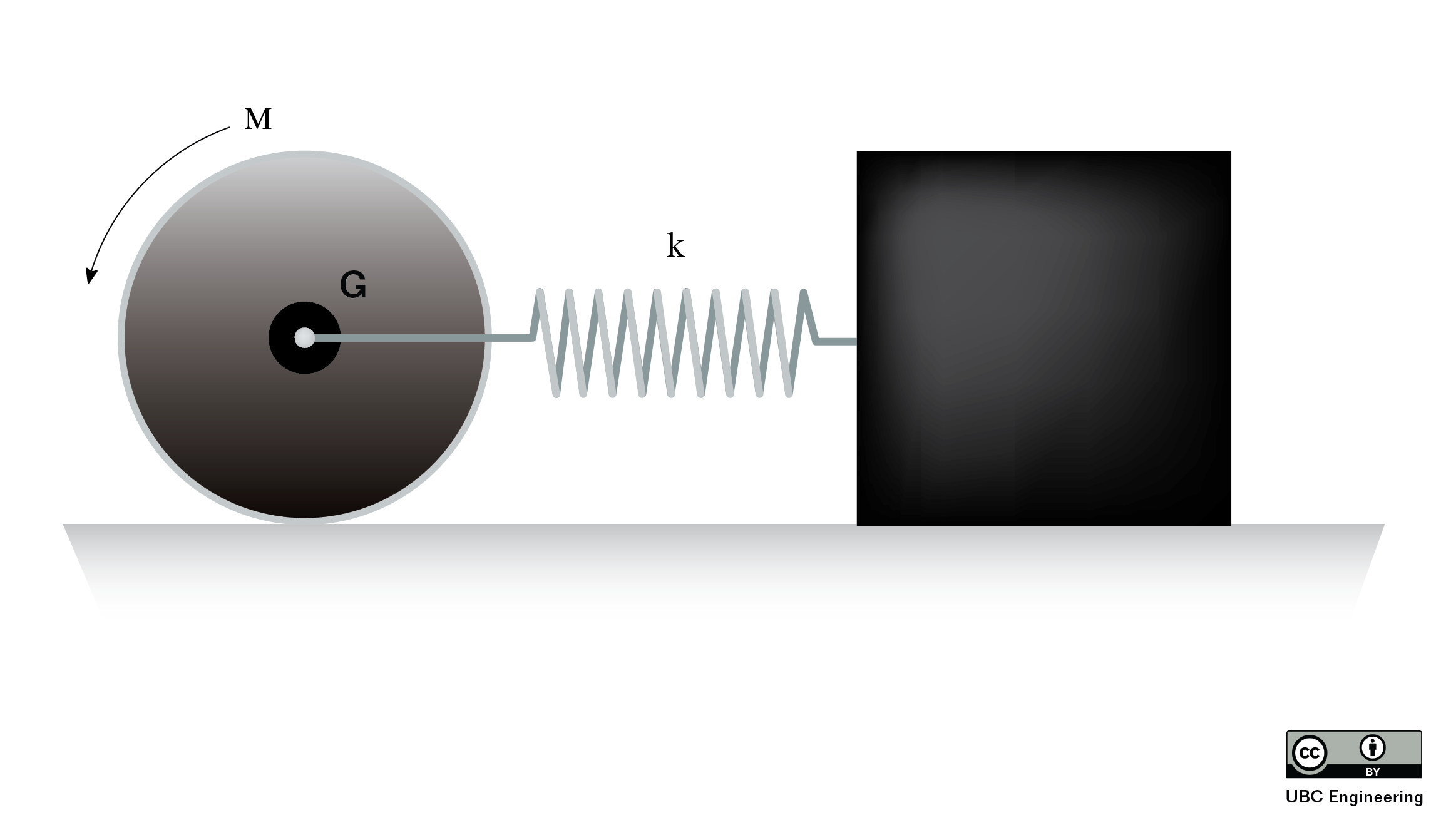### Question 5:

For a summer, you’ve taken a job at your uncle’s auto shop. You pull on the left side of a chain wrapped around a pulley with a force of F=50 N. The pulley has a mass m=20 Kg and a radius r=0.2 m. If the chain has a mass of 3.4 Kg/m, determine the angular velocity of the pulley after it has rotated θ=90 degrees. There is lA=3 m of chain hanging off the left side and lB=2 m hanging off the right side of the pulley. Assume the chain does not slip and that the system was released from rest just before you pulled on it. Assume the pulley can be modeled as a disk.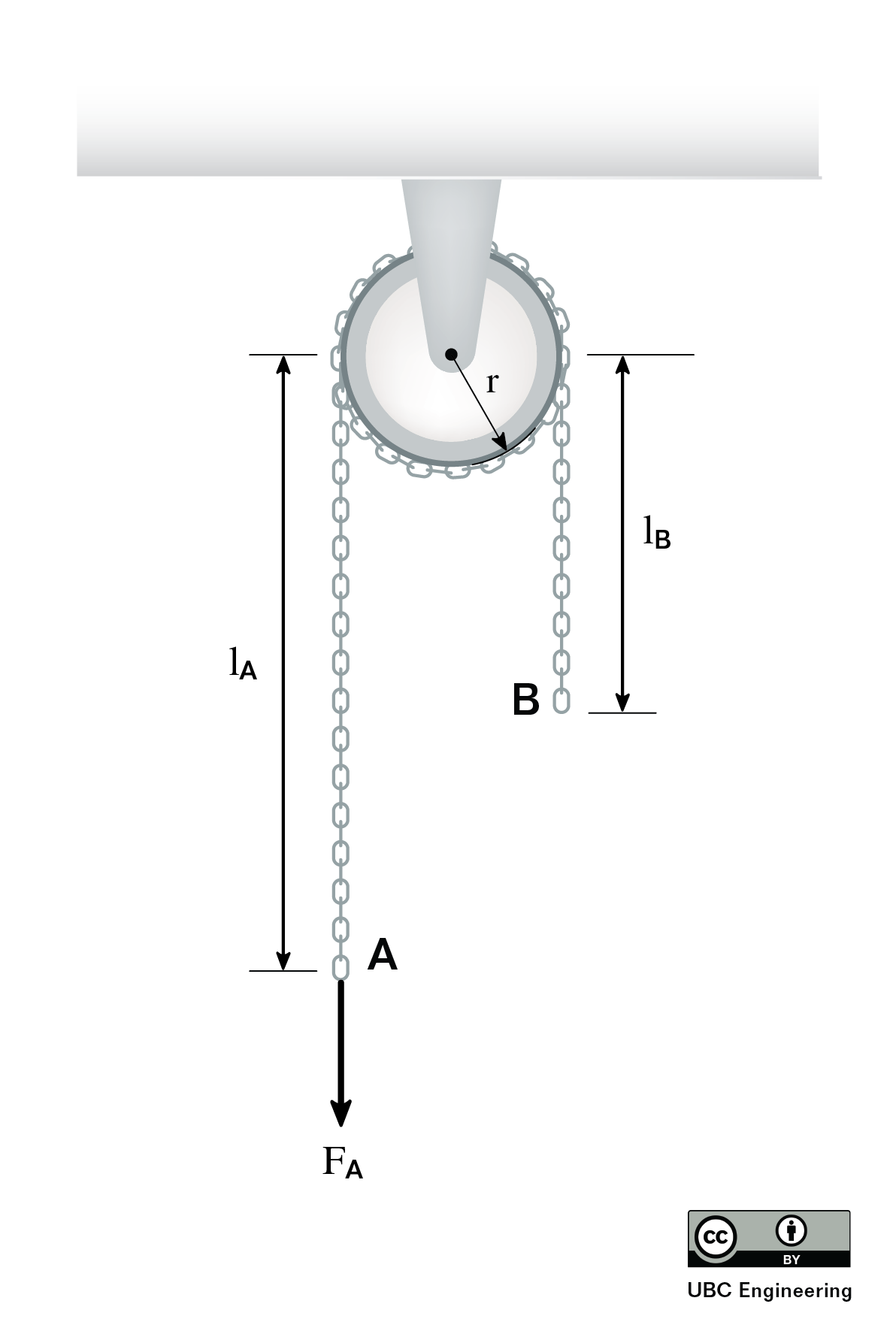### Question 6:

A 30Kg sheet of ice with length l=1.5 m at an angle θ=30 degrees has a force F=650 N applied at B. Determine the magnitude of the angular velocity of the plank when it reaches a vertical position. Assume the sheet acts like a thin plate and that contact between the sheet and all surfaces is frictionless.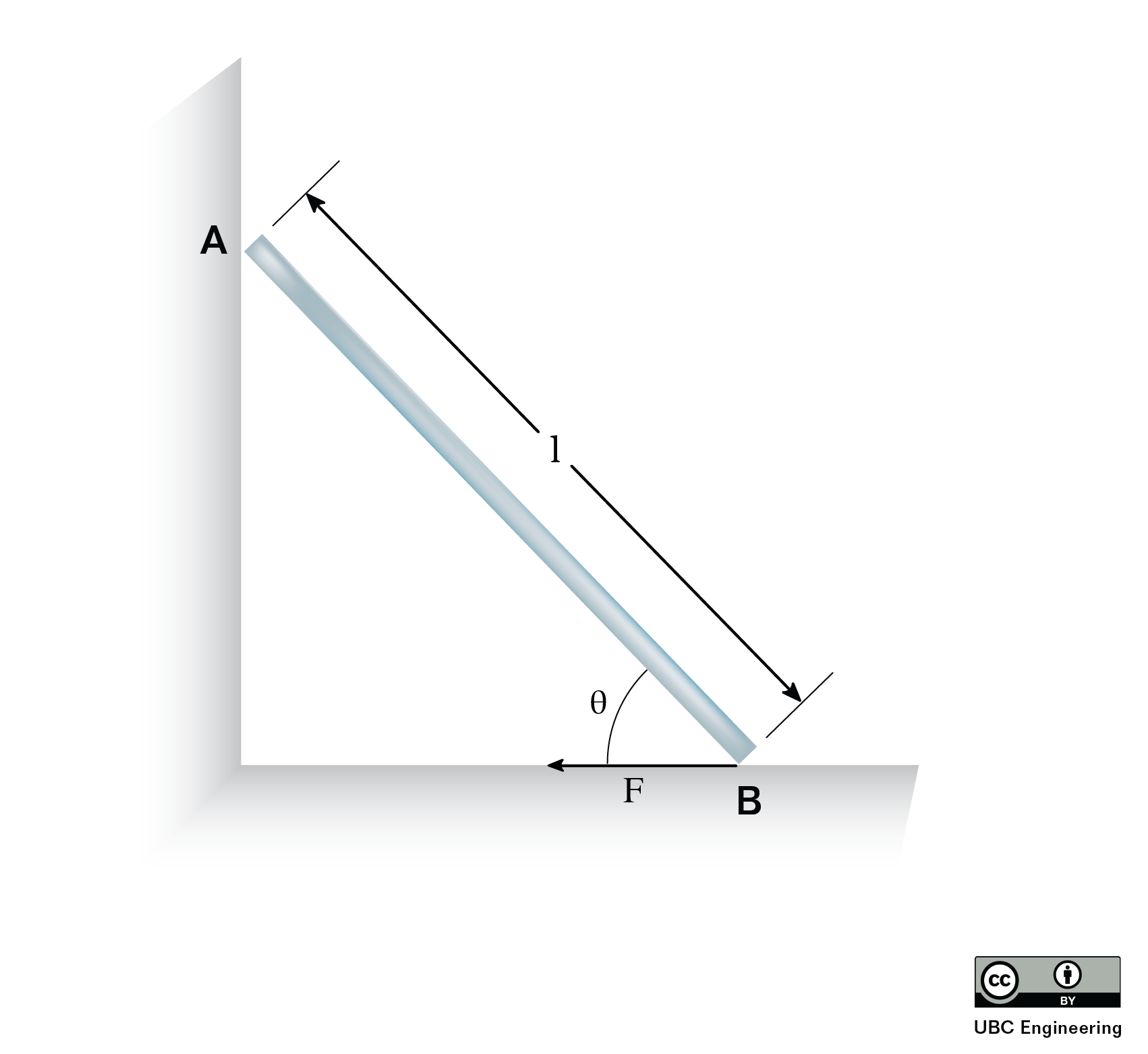### Question 7:

A reel of mass 15 kg, resting on two rollers is initially at rest when a force of P = 400 N is applied to a rope attached to the reel. Given that r1 = 0.2 m, r2 = 1 m, and the radius of gyration of the reel is 0.6 m, how many revolutions must the wheel complete to achieve a final angular velocity of 30 rad/s? (Assume no energy is lost due to friction and neglect the mass of the rope and the two rollers)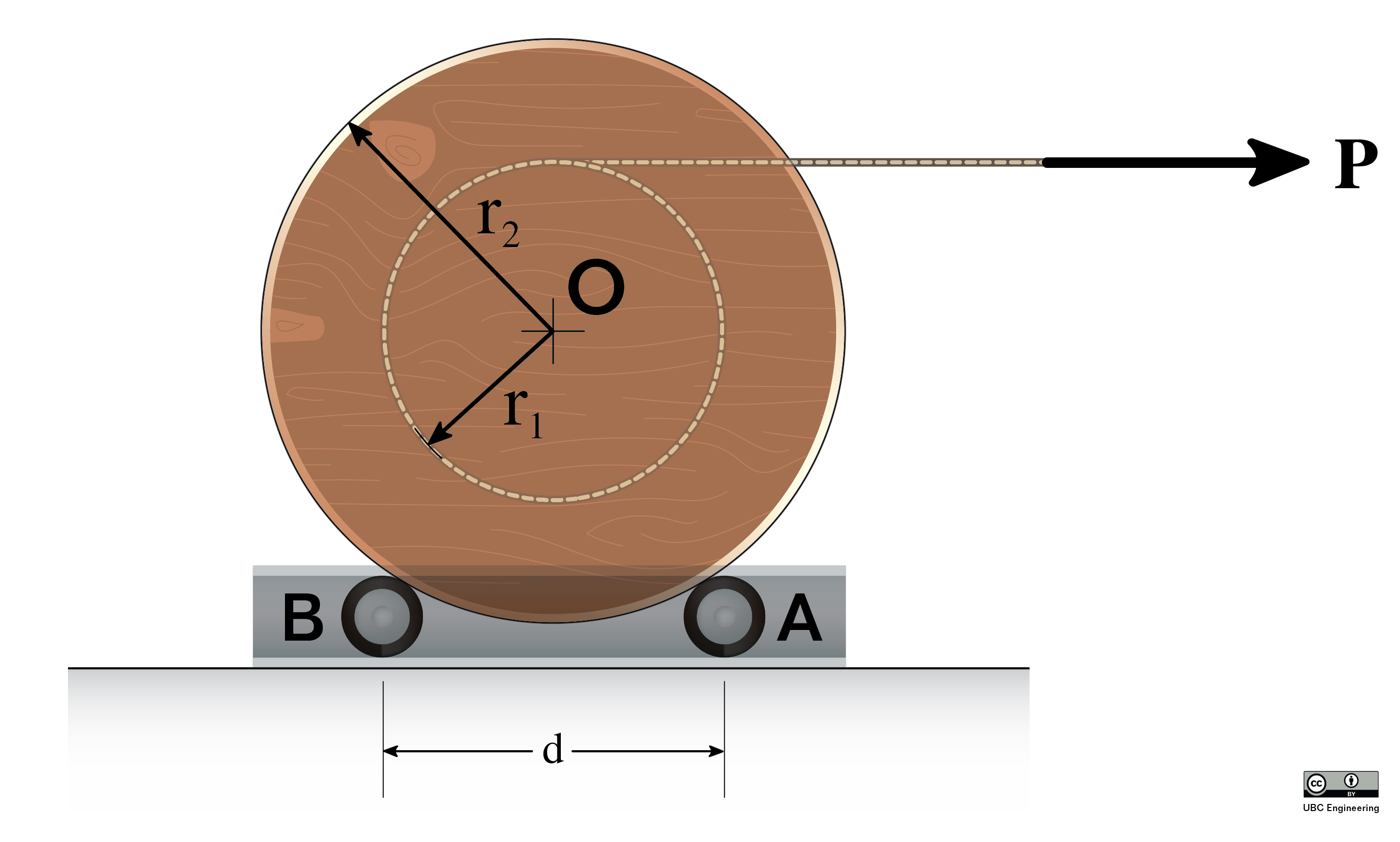### Question 8:

A 16 kg half cylinder is placed on a hard, flat surface as shown below and released from rest. What will the maximum angular velocity be as it rocks back and forth?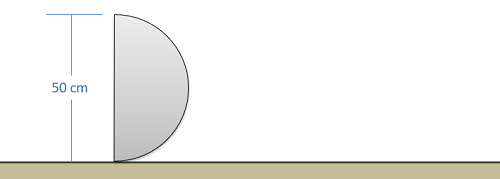### Question 9:

A mechanism consists of two, 3 kilogram wheels connected to a 2 kilogram bar as shown below. Based on the dimensions in the diagram, what is the minimum required initial velocity for the wheels to ensure the mechanism makes it all the way through one rotation without rocking backwards?